Next: Electron Scattering Up: Propagator Methods Previous: Propagator for the Dirac

# S-Matrix

We first consider the nonrelativistic case and then the relativistic case. In order to define properly the scattering problem, there should be no interaction at the initial time, so that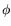is a solution of the free-particle equation which incorporates the required initial conditions.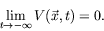(6.85)

The exact wave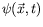becomes the incoming wave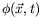in the limit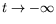: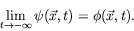(6.86)

We are primarily interested in the form of the scattering wave as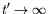. In this limit the particle emerges from the interaction region and again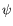becomes a solution of the free-particle equation: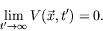(6.87)

All information about the scattered wave may be obtained from the probability amplitude for the particle to arrive in various final free states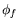asfor a given incident wave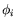. The probability amplitude for a give pair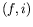is an element of the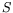-matrix (or scattering matrix)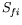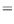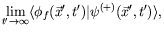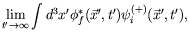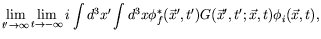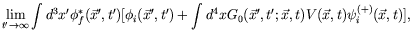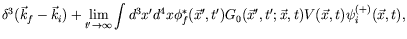(6.88)

where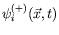is the solution of the wave equation (6.14) which reduces to a plane wave of momentum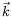, as. The superscript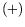over theis meant to express the fact that we are dealing with a wave which propagates into the future. We may expand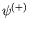in a multiple scattering series by iteration of equation 6.14 and thus express the-matrix in a multiple scattering series. The-matrix is the matrix which transforms the incoming state into the outgoing scattering state.

Finally, if we insertfrom the iterated solution of equation 6.27, we get an expression for the-matrix in terms of multiple scattering events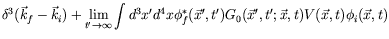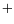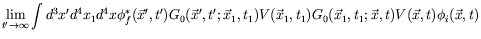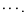(6.89)

The first term (the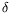-function) does not describe scattering but characterizes the particle flux without scattering. The second term represents single scattering, the third term double scattering, etc. They are coherently summed to give the total-matrix element.

For the relativistic case, the-matrix elements are defined in the same manner as in the nonrelativistic case. Terming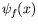the final free wave with the quantum numbers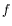that is observed at the end of the scattering process, we infer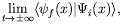(6.90)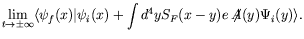Here the limit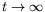is understood ifdescribes an electron andifdescribes a positron, since the latter is considered a negative-energy electron moving backward in time.

For electron scattering we have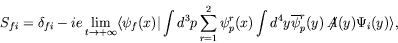(6.91)

while positron scattering is described by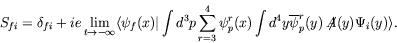(6.92)

The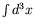integral implied by the brackets projects out just that state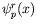whose quantum numbers agree with. All other terms of the integral-sum,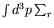, do not contribute. For electron scattering this yields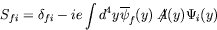(6.93)

and a similar expression for positron scattering. Both results can be combined by writing (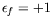for positive-energy waves in the future and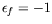for negative energy waves in the past)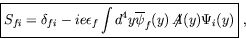(6.94)

where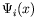stands for the incoming wave, which either reduces at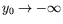to an incident positive-frequency wave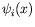carrying quantum number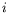or at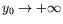to an incident negative-frequency wave propagating into the past with quantum number, according to Stückelberg-Feynman boundary conditions.

Equations 6.82 and 6.94 contain the rules for calculating the pair production and annihilation amplitudes, as well as, the ordinary'' scattering process. In practice we shall usually calculate only the first non-vanishing contribution to thematrix for a given interaction. The validity of this procedure depends on the weakness of the interaction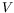and the rapid convergence of this series in powers of the interaction strength.

The application of the theory to special systems is considerably simplified by the symmetry properties of the-matrix under charge conjugation. The matrix elements of charge conjugation processes are equal. The photon-positron scattering cross-section is therefore equal to the photon-electron scattering cross-section. The Møller cross-section for electron-electron collisions is also valid for positron-positron collisions. These examples show that the charge symmetry of the theory reduces considerably the number of processes which must be calculates.

Arrows on the world line in a Feynman diagram keep track of entry and exit at each vertex. An arrow forward in time implies positive energy, while an arrow backward in time signifies negative energy There is no distinction between particle and antiparticle propagators since the Feynman prescription does both simultaneously.

SubsectionsNext: Electron Scattering Up: Propagator Methods Previous: Propagator for the Dirac
Douglas M. Gingrich (gingrich@ ualberta.ca)
2004-03-18